﻿ How the Default Probability is Defined by the CreditRisk+Model?Publications are Open
Access in this journal
Article Versions
Export Article
• Normal Style
• MLA Style
• APA Style
• Chicago Style
Review Article
Open Access Peer-reviewed

How the Default Probability is Defined by the CreditRisk+Model?

International Journal of Global Energy Markets and Finance. 2018, 1(1), 21-25. DOI: 10.12691/ijgefm-1-1-4
Published online: April 24, 2018

Abstract

The aim of this paper is to investigate theoretically one of the current models of credit portfolio management. There are currently three types of models to consider the risk of credit portfolio: the structural models (Moody's KMV model and CreditMetrics model) also defined by the models of the value of the firm, reduced form models also defined by models with intensity models (the actuarial models) and the econometric models (the Macro-factors model). The development of the three types of models is based on a theoretical basis developed by several researchers. The evolution of their default frequencies and the size of the loan portfolio are expressed as functions of macroeconomic and microeconomic conditions as well as unobservable credit risk factors, which explained by other factors. We developed this paper to explain the different characteristics of the CreditRisk+ models. The purpose of this model is to calculate the default probability of credit portfolio.

1. Introduction

The problem of evaluation of the failure probability of any borrower was the center of the bankers as soon as they began to lend some money. The quantitative modeling of the credit risk for a debtor is rather recent in fact. Besides, the modeling of the credit risk associated with instruments of a portfolio of credit such as, the loans, the pledges, the guarantees and the by-products (who constitute a recent concept).

A certain number of models were developed, including at the same time the applications of property developed for the internal custom by the financial institutions, and the applications intended for the sale or for the distribution 1.

The big financial institutions recognize his necessity, but there is a variety of approaches and rival methods. There are three types of models of credit portfolio in the course of use at present 2:

• The structural models: there are two models of management of credit portfolio who are supplied in the literature: Moody's KMV model (Portfolio Model) and CreditMetrics model by JPMorgan.

• The Macro-factors model (Econometric model): The Credit Portfolio View model introduces in 1998 by Mckinsey.

• The actuarial models CSFP (Credit Suisse First Boston): this model (CreditRisk+) is developed in 1997.

The main idea for this study is to answer the question follows: How the default probability is defined by the CreditRisk+ model?

Then, the organization of this paper is as follows. In section 2, we present the CreditRisk+ model and we define the forces and the weaknesses of this model. Finally, we conclude in section 3.

2. The Model CSFP: CREDIT RISK+ MARKET RISK

Since 1990s, Credit Suisse First Boston (CSFB) has developed new methods of risk management. In 1993, the credit Swiss Group launched, in parallel of an important project which aims at modernizing its credit risk management and by using the expertise of CSFB, new one management tool of the credit portfolio in the future. In December, 1996, Credit Suisse Group presented the CreditRisk+ model as being a model of the credit portfolio management.

The structural models present an inconvenience concerning the default. These models suppose that the default cannot have arisen by surprise because the market value of assets is supposed to follow a continuous process of distribution. In this aligned, a process of Fish was used in the actuarial models the purpose of which is to model the unpredictable character of the emergence of the default what is developed in the model CreditRisk+.

CreditRisk+ is a model with intensity is which presents no hypothesis on the causes of failure of a company. It is model statistical of the default of credit risk which makes no claim about the causes of the default. This approach is similar to that of the management of the market risk, in which no attempt is made model the causes of the movements of market prices. This model does not consider the consequences of a deterioration of the quality of the quality of the counterparty.

So, the number of failures in a credit portfolio during the given period justifies itself by a process of Fish. CreditRisk+ uses a methodology based on techniques and quantitative methods. The present model is based on an actuarial calculation to determine and present the distribution of the losses of a credit portfolio.

• The CreditRisk+ presents four hypotheses:

• Every individual credit presents only two possible states: failures or no failures.

• The default probability of an individual credit is low.

• The default probability for a big group of borrowers is very low.

• The number of default over a period is independent from that of any other period.

By basing itself on these hypotheses, the probability distribution of the number X of defaults over a given period (one month or one year for example) can be represented by using the law of Fish of average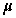and of standard deviation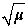:Where,is the average of the number of default a year.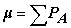Where,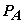indicate the default probability of the obligor A.

The annual number of the defaults, n, is a stochastic variable of averageand a standard deviation. According to CreditRisk+, the calculation of the distribution of the losses requires the use of an approach by bonds; that is issued in a portfolio are grouped and collected by edge of exposure.

The process of determination of the distribution of the losses of a portfolio is constituted by three stages:

• The determination of the generative function of probability for every bond.

• The diversion of the generative function of probability for the whole portfolio.

• The determination of the distribution of the losses for the whole portfolio.

The distribution of the losses of default for a portfolio is diverted in two stages as the watch represents it below (Figure 1).

Until here, we suppose that the distribution of fish allows moving closer to the distribution of the number of the events of defect. Then, we should expect that the standard deviation of the default rate is approximately equal to the square root of the average.

In case of defect of an obligor, the counterparty incurs a loss equal to the quantity possessed by the obligor less a quantity of restoring. In CreditRisk+ the exposure for every obligor is adjusted by the rate planned by restoring, to calculate the loss of default. These adjusted exposures are exogenous in the model, and are independent of the market risk and minimize the risk.

To divert the distribution of loss for a diversified portfolio, the losses are divided into bands with the level of the exposure in every band.

To analyze the distribution of the resultant losses of the whole portfolio, presenting us the default probability expressed by the function defined in terms of variables auxiliary z by respecting itself the following approach of the formulation of the generative function:

We considered X a whole and positive random variable. The generative function of X is the whole series: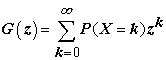Where P(X=k) is the probability that the random variable X takes the value k. to obtain P(X=k) from the generative function G(z), we use the following formula: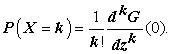In that case, the generative function associated among default X arisen among all the bonds of a portfolio is given by the expression below: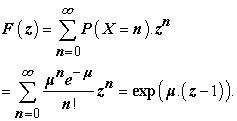View option
• Figure 1. CreditRisk+ risk measurement framework 

This function can be written as follows:Where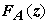indicate the generative function of a portfolio constituted by a single bond of the issuer A.

So, every portfolio consists of m identical bond of exposure of indications j (j = 1, 2, m).

Every bond is characterized by:Thus implies that:With,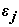indicate the expected average loss expressed in multiple of a standard exposure L,indicate the expected number of defaults which is a known value and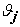indicate the exposure expressed in multiple of L in the band j.

In that case, the inputs of the model to be developed are: the individual exposure L and the probability of defaultfor the issuer (debtor) A. Then, the loss hoped for the debtor A is expressed as follows:The expression above is obtained when the expected loss is expressed in units of L. So, the expected lossfor the bond j is given then as follows: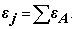In this perspective, the expected number of defectsfor each of the indicated bond j is then given by: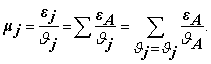Thus, the number of waited defects totalfor them m bond is expressed as followsThe expression of the generative function of the included losses is obtained by: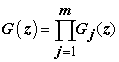Thus: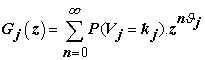Where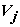represents the amount of the losses of the bond j and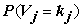indicates the probability of the loss.

Furthermore, we have:Thus we obtain:And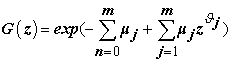Then, if we put: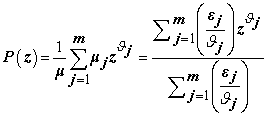Then, the generative function of the included losses can be written in the following way:Where from, we can obtain the distribution of the losses of the total portfolio of an amount (n*L) as follows: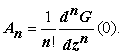Land us note in that case that,can be calculated in continuous by basing itself on the following formula and under the hypothesis according to whichis constant.

Where from we obtain: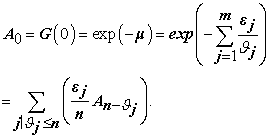The CreditRisk+ model considers that every sector is driven by a simple fundamental factor. This factor explains the variability of the rate of average defect measured for this sector. The fundamental factor influences the rate of defects planned in the concerned sector which is modeled by a random variable of averageand of standard deviationindicated for every sector.

The standard deviation reflects the degree to which, in all the probability of default, the obligors in the portfolio are exposed are more or less that their levels of the average. By continuing this analysis, the model CreditRisk+ bases on the hypothesis thatis constant. So, by basing itself on the distribution of Fish of parameterthe probability of failures are underestimated. In that case, it is necessary to take into account the existence of an average number of variable failures.

In this alignment, the parameteris considered as being a stochastic variable and depends on characteristics of the sector. In fact, and according to the CreditRisk+ model, a sector is considered as being a sand of credits the rates of failure of which are subjected to the same influences. In the CreditRisk+ model, every portfolio is divided into sectors indicated by k with 1 ≤ k ≤ K.

In particular, for every sector k, we introduce one random variablewhich represents the average number of defaults in this sector. The average number of the defects is equal in.

So, the hope offor the sector k is notedand its standard deviation is equal in. In this frameis calculated as follows: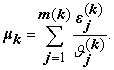In the case thatis no constant; the generative function of the number of defaults is given by:And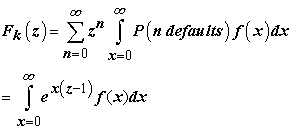Whereindicates the density of the variable.

The continuation of the calculations is conditioned by the presence of a nature of distribution given in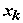. In the CreditR is k+ model, the choice is fixed to a distribution Gamma Г of average µ and of standard deviation. Thus we obtain:Where the Gamma function written as follows: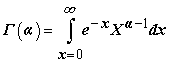For every sector k, we have two parameters of Gamma function to be estimated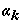and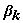.

Thus: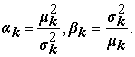By substituting and by basing itself on the definition of the Gamma function, we obtain then: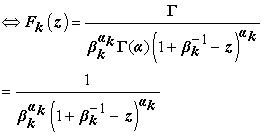After this simplification, the generating function of the distribution of the probabilities of default for the sector K is given by the following expression: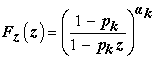Thus: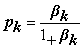After the determination of the number of defaults in a portfolio, one goes in what follows to present the generating function of the losses incorporated in a portfolio functions written is the following;So: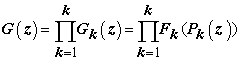Where the polynomial functionis written as follows: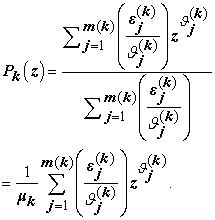One can deduce the expression from the generating functionwhich is written in the following way:In this respect, we can deduct the distribution of the losses of portfolios from the An which is given by:So, in caseverify the following relation: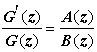Where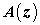and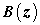are two polynomials of the following shape: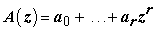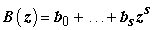Thus, the coefficientsverify the relation of following recurrence: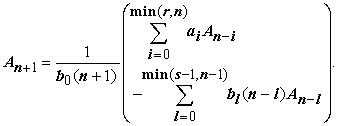This relation is applied knowing that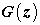verify the following condition: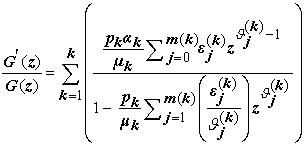Generally, the CreditRisk+ model is based on mathematical techniques in the modeling of the distribution of the losses in the field of the banking activities and of the insurance. The behavior of common default of the borrowers is incorporated by treating the rate of default as being a common random variable for multiple borrowers. So, the borrowers are assigned among the sectors among which each has a rate of average default and a volatility of rate of default. The volatility of rate of default is the standard deviation which would be observed on a portfolio of infinitely diversified homogeneous credit.

The forces and the weaknesses relative to the CreditRisk+ model are presented in the table below 3:

3. Conclusion

In this study, we expose a theoretical approach’s concerning the model of management of credit portfolio by the actuarial models CSFP (Credit Suisse First Boston).

However, structural models are based on option theory and capital structure the company. On econometric models, they link the probability fault of the company to the state of the economy. The probability of failure depends in these models of macroeconomic factors such as unemployment, the rate of increase GDP, the interest rate long-term. Moreover, in the CreditRisk+ models, the probability of default varies over time.

References

  Koyluoglu, H., and Hickman, A. (1998). Reconcilable differences, Risk, 11(10): 56-62. In article  Crouhy, M., Galai, D. and Mark, R. (2000). A comparative analysis of current credit risk models. Journal of Banking & Finance, (24): 59-117. In article View Article  Hamisultane, H. (2008). Modèles de gestion du risque de crédit. Investment System R&D, Document n°1. In articleThis work is licensed under a Creative Commons Attribution 4.0 International License. To view a copy of this license, visit http://creativecommons.org/licenses/by/4.0/